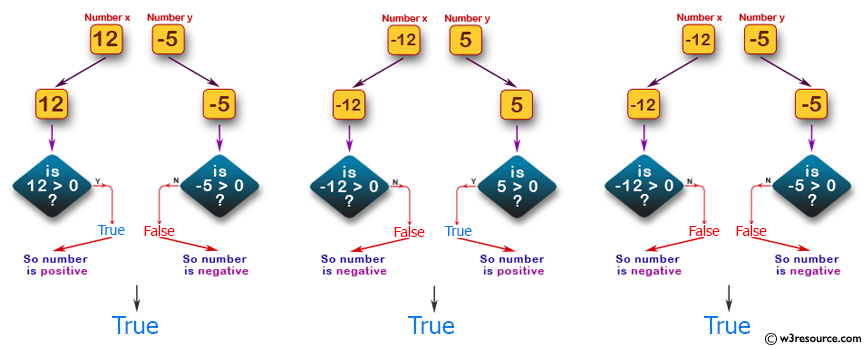﻿ Swift Basic Programming Exercise: Accept two integer values and return true if one is negative and one is positive - w3resource# Swift Basic Programming Exercise: Accept two integer values and return true if one is negative and one is positive

## Swift Basic Programming: Exercise-4 with Solution

Write a Swift program to accept two integer values and return true if one is negative and one is positive. Return true only if both are negative.

Pictorial Presentation:Sample Solution:

Swift Code:

``````func test_num(x: Int, y: Int) -> Bool {
if x > 0 && y < 0
{
return true
}
else if x < 0 && y > 0
{
return true
}
else if x < 0 && y < 0
{
return true
}
else
{
return false
}
}

print(test_num(x:12, y:-5))
print(test_num(x:-12, y:5))
print(test_num(x:-12, y:-5))
print(test_num(x:12, y:5))

```
```

Sample Output:

```true
true
true
false
```

Swift Programming Code Editor:

Improve this sample solution and post your code through Disqus

What is the difficulty level of this exercise?

﻿